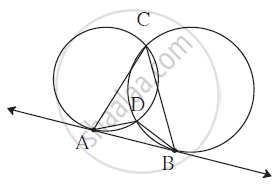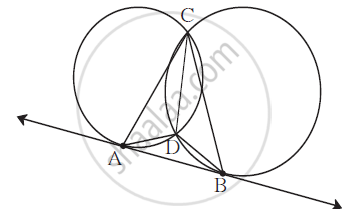SSC (English Medium) Class 10th Board ExamMaharashtra State Board
Share

# Two Circles Intersect Each Other at Points C and D. Their Common Tangent Ab Touches the Circles at Point a and B. Prove that :∠ Adb + ∠ Acb = 180° - SSC (English Medium) Class 10th Board Exam - Geometry

ConceptTangent Properties - If Two Circles Touch, the Point of Contact Lies on the Straight Line Joining Their Centers

#### Question

Two circles intersect each other at points C and D. Their common tangent AB touches the circles at point A and B. Prove that :
∠ ADB + ∠ ACB = 180°#### SolutionDraw seg CD.
∠ DAB = ∠ ACD .... (1) Tangent secant angle theorem
∠ DBA = ∠ DCB .... (2) Tangent secant angle theorem
From (1) and (2)
∠ DAB + ∠ DBA = ∠ ACD + ∠ DCB
Now, ∠ ACB = ∠ ACD + ∠ DCB ...... (3)